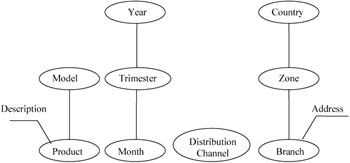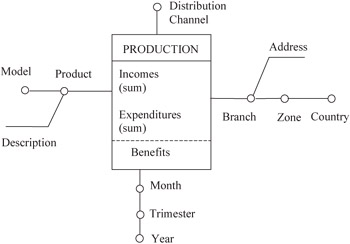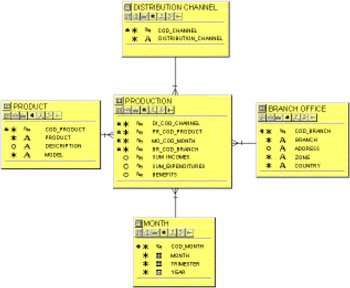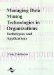# Example

 < Day Day Up >

In the following sections, we will see a small example. First, we will obtain the IDEA conceptual schema. Next, we will translate it to specific relational and multidimensional schemes. Conceptual schema should be obtained in the ASI-MD 3 activity, "data warehouse conceptual modeling." Translation into logical schemes should be done in activity DSI-MD 2, "multidimensional logical design " (in the case of MOLAP implementation) or DSI-MD 3, "multidimensional relational logical design" (in the case of ROLAP implementation).

### Conceptual Modeling

Let's suppose that we are modeling the results obtained by the sales of some products by a company. We are interested in analyzing the products sold, the date, the branch office, and the distribution channel used for the sale.

The purpose of the first tasks of ASI-MD 3 activity is to obtain a preliminary structure of the conceptual schema. So, in those tasks we should detect the four dimensions and a general overview of the dimensioned facts (incomes, expenditures, and benefits). Next, it is important to make a detailed study of the dimensions (perhaps new dimensions could be detected). We obtain then a detailed description of hierarchies, dimension attributes, and description attributes. Aggregation functions between dimension domains should also be defined. Figure 6 shows the dimensions detected. Two of them are very common: time (in this case, month-trimester-year) and geography (branch-zone-country). There are also two description attributes, one of them associated to the branch dimension attribute (which should contain the branch address), and the other associated to the product dimension attribute (containing a description of such product). As we can see, one of the dimensions (distribution channel) has only one dimension attribute.Figure 6: Dimensions detected

The next step is to detail the fact schemas. We must specify synthesis attributes, synthesis functions applied on them, and methods. In this case, we have two synthesis attributes (incomes and expenditures). The aggregation function in both cases is sum. Besides that, we have a method (benefits), defined by sum of incomes minus sum of expenditures. Figure 7 shows the resulting conceptual schema.Figure 7: Conceptual schema

### Logical Design: Relational

In DSI-MD, process transformation from conceptual into logical multidimensional or relational schema is carried out. In the relational case, the conceptual schema is translated into a star schema (Kimball, 1996; Kimball et al., 1998). Every dimension is transformed into a dimension table. Physical design should be done taking into account the characteristics of the specific relational product, such as bitmap indices, table partitions, materialized views, etc.

So, every fact schema is transformed into a fact table, and every dimension into a dimension table. The fact table primary key is composed of the foreign keys of the dimension tables. Every synthesis attribute without synthesis function is transformed into columns of the fact table (in this case, data have not been summarized from the operational databases). If synthesis functions exist, every function is transformed into a column, which will store the result of applying such function to operational data. A method is also transformed into fact table columns, or views (perhaps materialized views) obtained from synthesis attributes and functions. In the example, the benefits method should be transformed into a column. Figure 8 shows the relational schema corresponding to the conceptual schema of Figure 7 in Oracle Designer/2000 format.Figure 8: Star schema

### Logical Design: Multidimensional

Because there is not any standard logical multidimensional model, transformation rules in this case have to be based on specific products. Next, we show a transformation into Oracle Express, one of the most used multi dimensional DBMS.

Dimension attributes of the IDEA model can be directly transformed into express dimensions by means of the command Define name dimension type [width number]. Description attributes must also be transformed into dimensions. In express we can not distinguish between description and dimension attributes. Aggregation functions are transformed into express relations. We have to make correspondences between dimension values affected. Synthesis attributes with no associated function are transformed into express variables. If there is a synthesis function associated with the attribute, every function is transformed into a variable, using the command Define name variable type <dimensions>. IDEA methods can be transformed into express formulas of variables, depending on efficiency issues, storage space, and other physical design criteria. If we choose a variable, pre-calculated data are physically stored (so response times are reduced). If we choose a formula, data are calculated "on the fly" (so response times are worse, and storage space is reduced). The command for defining a formula is Define name formula expression type <dimensions>. Finally, a multidimensional schema should be transformed into an express database by means of the command Database create name.

 < Day Day Up >Managing Data Mining Technologies in Organizations: Techniques and Applications
ISBN: 1591400570
EAN: 2147483647
Year: 2003
Pages: 174